## 15.5.1 Using the P. Hall Basis

The steering method presented in this section is due to Lafferriere and Sussmann . It is assumed here that a driftless control-affine system is given, in whichis a Lie group, as introduced in Example 15.15. Furthermore, the system is assumed to be STLC. The steering method sketched in this section follows from the Lie algebra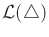. The idea is to apply piecewise-constant motion primitives to move in directions given by the P. Hall basis. If the system is nilpotent, then this method reaches the goal state exactly. Otherwise, it leads to an approximate method that can be iterated to get arbitrarily close to the goal. Furthermore, some systems are nilpotentizable by using feedback .

The main idea is to start with (15.53) and construct an extended system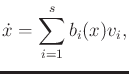(15.114)

in which each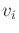is an action variable, and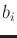is a vector field in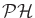, the P. Hall basis. For every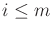, each term of (15.114) is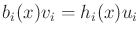, which comes from the original system. For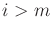, eachrepresents a Lie product in, andis a fictitious action variable. It is called fictitious because the velocity given byforcannot necessarily be achieved by using a single action variable of the system. In general,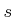may be larger thanbecause at each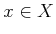a different subset ofmay be needed to obtainindependent vectors. Also, including more basis elements simplifies some of the coming computations.

Example 15..21 (Extended System for the Nonholonomic Integrator)   The extended system for the nonholonomic integrator (15.83) is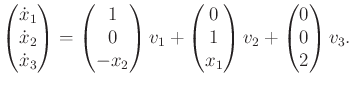(15.115)

The first two terms correspond to the original system. The last term arises from the Lie bracket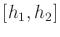. Only one fictitious action variable is needed because the three P. Hall vector fields are independent at every.

It is straightforward to move this system along a grid-based path in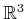. Motions in the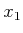and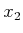directions are obtained by applying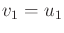and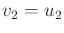, respectively. To move the system in the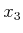direction, the commutator motion in (15.71) should be performed. This corresponds to applying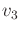. The steering method described in this section yields a generalization of this approach. Higher degree Lie products can be used, and motion in any direction can be achieved.Suppose some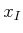and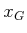are given. There are two phases to the steering method:

1. Determine an action trajectory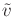for the extended system, for which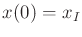and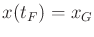for some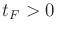.
2. Convertinto an action trajectory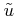that eliminates the fictitious variables and uses the actual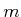action variables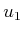,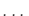,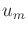.
The first phase is straightforward. For the extended system, any velocity in the tangent space,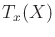, can be generated. Start with any smooth path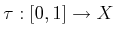such that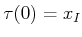and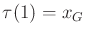. The velocity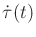along the path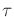is a velocity vector in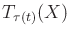that can be expressed as a linear combination of the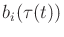vectors using linear algebra. The coefficients of this combination are thevalues. The second phase is much more complicated and will be described shortly. If the system is nilpotent, thenshould bring the system precisely fromto. By the way it is constructed, it will also be clear how to refineto come as close as desired to the trajectory produced by.

Subsections
Steven M LaValle 2020-08-14